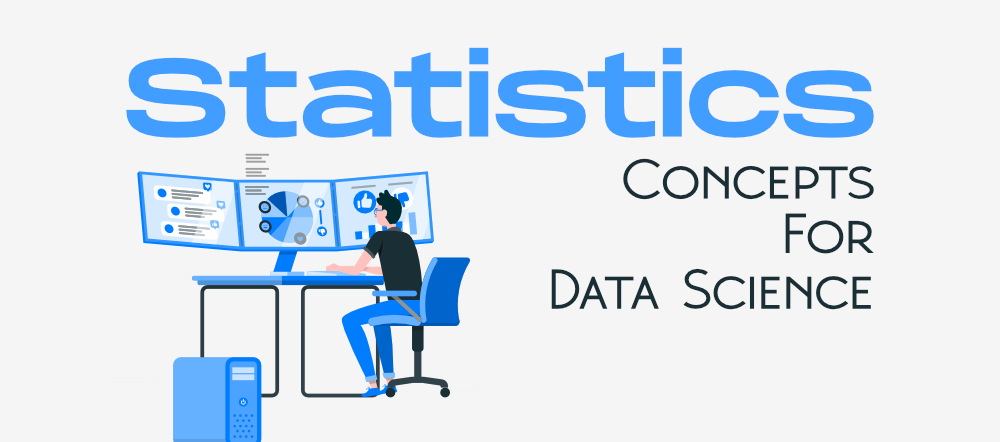# 7 Basic Statistics Concepts For Data Science

• Difficulty Level : Basic
• Last Updated : 23 Nov, 2021

Data Scientist is one of the most lucrative career options that offers immense job satisfaction, insanely high salary, global recognition, and amazing growth opportunities. Further, this profession offers an astonishing job satisfaction rating of 4.4 out of 5. As per the Harvard Business Review, Data Scientist is defined as the most desirable profession of the 21st century. Machine Learning and Statistics are the two core skills required to become a data scientist.Statistics is like the heart of Data Science that helps to analyze, transform and predict data. So if you are willing to ace your career in this astonishing domain then it is really important to get yourself familiar with all the relevant Statistics topics for data science. Statistics is an extremely wide field and determining what you need to learn can be difficult. To make your learning experience smooth we can help you. In this blog, we will discuss the seven basic Statistics Concepts for Data Science. The topics covered in this blog will build the basic foundation of your statistical skills.

So let’s get started:

### 1. Descriptive Statistics

It is used to describe the basic features of data that provide a summary of the given data set which can either represent the entire population or a sample of the population. It is derived from calculations that include:

• Mean: It is the central value which is commonly known as arithmetic average.
• Mode: It refers to the value that appears most often in a data set.
• Median: It is the middle value of the ordered set that divides it in exactly half.

### 2. Variability

Variability includes the following parameters:

• Standard Deviation: It is a statistic that calculates the dispersion of a data set as compared to its mean.
• Variance: It refers to a statistical measure of the spread between the numbers in a data set. In general terms, it means the difference from the mean. A large variance indicates that numbers are far apart from the mean or average value. Small variance indicates that the numbers are closer to the average values. Zero variance indicates that the values are identical to the given set.
• Range: This is defined as the difference between the largest and smallest value of a dataset.
• Percentile: It refers to the measure used in statistics that indicates the value below which the given percentage of observation in the dataset falls.
• Quartile: It is defined as the value that divides the data points into quarters.
• Interquartile Range: It measures the middle half of your data. In general terms, it is the middle 50% of the dataset.

### 3. Correlation

It is one of the major statistical techniques that measure the relationship between two variables. The correlation coefficient indicates the strength of the linear relationship between two variables.

• A correlation coefficient that is more than zero indicates a positive relationship.
• A correlation coefficient that is less than zero indicates a negative relationship.
• Correlation coefficient zero indicates that there is no relationship between the two variables.

### 4. Probability Distribution

It specifies the likelihood of all possible events. In simple terms, an event refers to the result of an experiment like tossing a coin. Events are of two types dependent and independent.

• Independent event: The event is said to be an Independent event when it is not affected by the earlier events. For example, tossing a coin, let us consider a coin is tossed the first outcome is head when the coin is tossed again the outcome may be head or tail. But this is entirely independent of the first trial.
• Dependent event: The event is said to be dependent when the occurrence of the event is dependent on the earlier events. For example when a ball is drawn from a bag that contains red and blue balls. If the first ball drawn is red, then the second ball may be red or blue; this depends on the first trial.

The probability of independent events is calculated by simply multiplying the probability of each event and for a dependent event is calculated by conditional probability.

### 5. Regression

It is a method that is used to determine the relationship between one or more independent variables and a dependent variable. Regression is mainly of two types:

• Linear regression: It is used to fit the regression model that explains the relationship between a numeric predictor variable and one or more predictor variables.
• Logistic regression: It is used to fit a regression model that explains the relationship between the binary response variable and one or more predictor variables.

### 6. Normal Distribution

Normal is used to define the probability density function for a continuous random variable in a system. The standard normal distribution has two parameters – mean and standard deviation that are discussed above. When the distribution of random variables is unknown, the normal distribution is used. The central limit theorem justifies why normal distribution is used in such cases.

### 7. Bias

In statistical terms, it means when a model is representative of a complete population. This needs to be minimized to get the desired outcome.

The three most common types of bias are:

• Selection bias: It is a phenomenon of selecting a group of data for statistical analysis, the selection in such a way that data is not randomized resulting in the data being unrepresentative of the whole population.
• Confirmation bias: It occurs when the person performing the statistical analysis has some predefined assumption.
• Time interval bias: It is caused intentionally by specifying a certain time range to favor a particular outcome.

These were some of the statistics concepts for data science that you need to work on. Apart from these, there are some other statistics topics for data science as well which includes:

• Central limit theorem